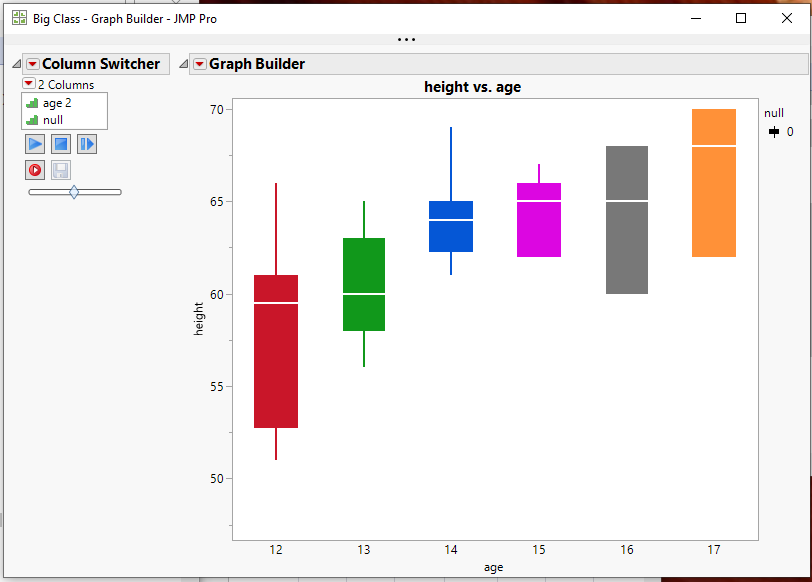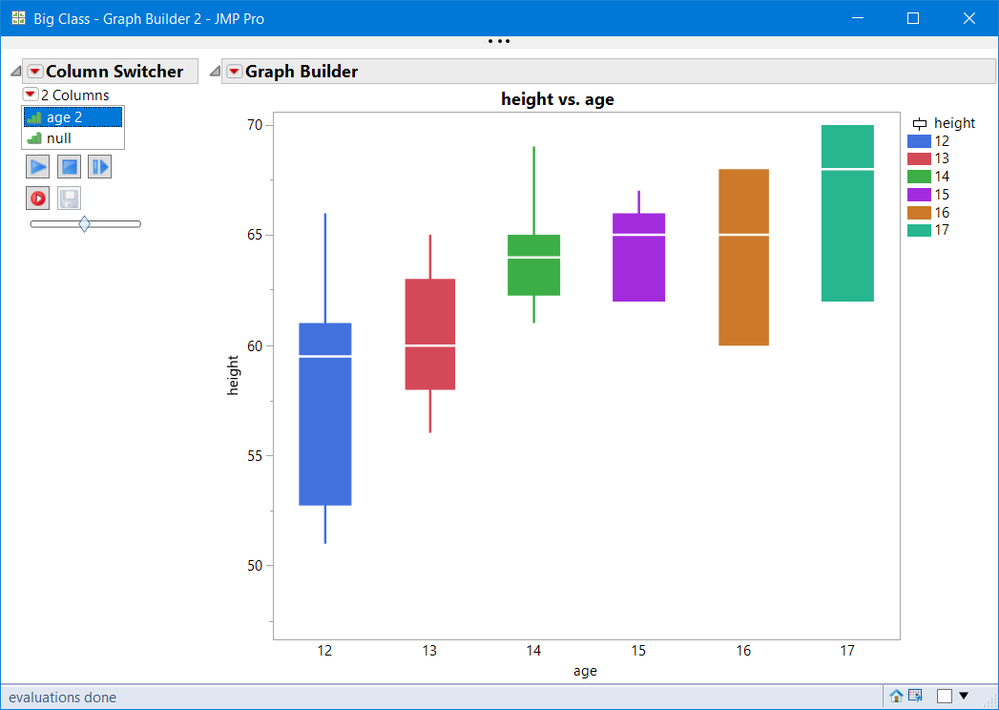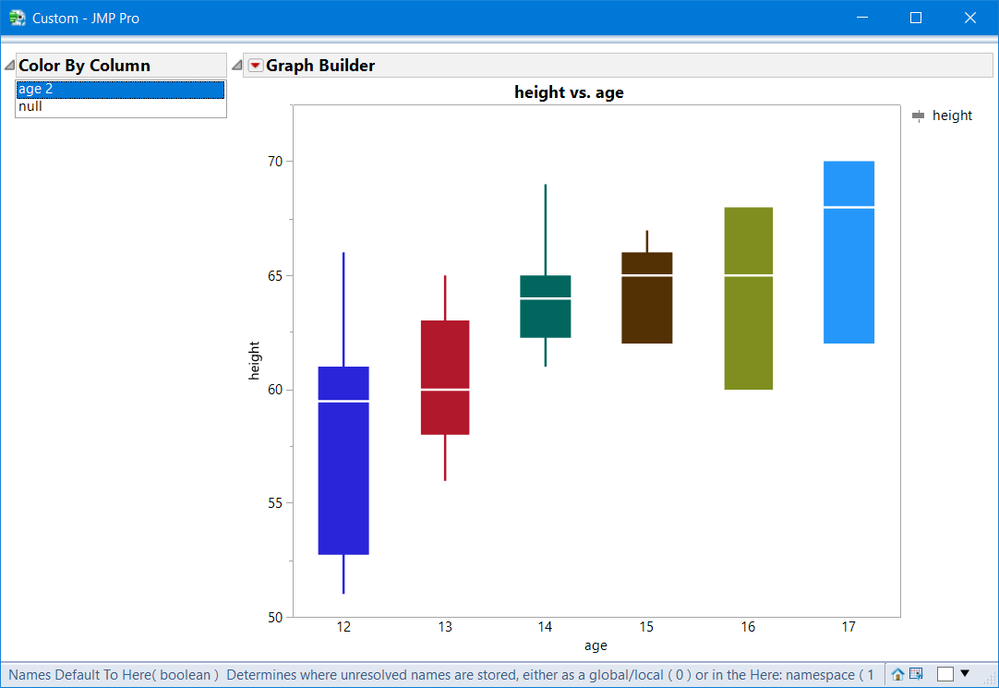Choose Language Hide Translation Bar
Highlighted

## Graph Builder Box Plot Color

On the Graph Builder Box Plot, it is possible to color each box using the "Overlay" feature, but this makes the graph slightly less readable by reducing the box width, which is unnecessary in some contexts.  Can JMP add a "Color" feature to the Graph Builder Box Plot?

I am using JMP 14.3.0.

``````Names Default To Here( 1 );
dt = Open( "\$SAMPLE_DATA/Big Class.jmp" );
dt << New Column( "age 2", Numeric, "Ordinal", Format( "Best", 12 ), Formula( :age ) );
dt << New Column( "null", Numeric, "Ordinal", Format( "Best", 12 ), Formula( 0 ) );

dt << Graph Builder(
Show Control Panel( 0 ),
Variables( X( :age ), Y( :height ), Overlay( :null ) ),
Elements( Box Plot( X, Y, Legend( 6 ), Box Style( "Solid" ) ) ),
Column Switcher( :null, {:age 2, :null} )
);``````
2 ACCEPTED SOLUTIONS

Accepted Solutions
Highlighted

## Re: Graph Builder Box Plot Color

You can interactively change the color of the box plots, by right clicking on the chart and selecting "Customize"Programmatically the script below was what the graph builder generated after I changed the color of the boxes

``````Graph Builder(
Show Control Panel( 0 ),
Variables( X( :age ), Y( :height ), Overlay( :null ) ),
Elements( Box Plot( X, Y, Legend( 6 ), Box Style( "Solid" ) ) ),
Column Switcher( :null, {:age 2, :null} ),
SendToReport(
Dispatch(
{},
"Graph Builder",
FrameBox,
{DispatchSeg(
Box Plot Seg( "Box Plot (12)" ),
{Line Color( "Medium Dark Red" ), Fill Color( "Medium Dark Red" ),
Fill( 0 )}
), DispatchSeg(
Box Plot Seg( "Box Plot (13)" ),
{Line Color( "Medium Dark Green" ),
Fill Color( "Medium Dark Green" ), Fill( 0 )}
), DispatchSeg(
Box Plot Seg( "Box Plot (14)" ),
{Line Color( "Medium Dark Blue" ), Fill Color( "Medium Dark Blue" ),
Fill( 0 )}
), DispatchSeg(
Box Plot Seg( "Box Plot (15)" ),
{Line Color( "Magenta" ), Fill Color( "Magenta" ), Fill( 0 )}
), DispatchSeg(
Box Plot Seg( "Box Plot (16)" ),
{Line Color( "Gray" ), Fill Color( "Gray" ), Fill( 0 )}
), DispatchSeg(
Box Plot Seg( "Box Plot (17)" ),
{Line Color( "Medium Light Orange" ),
Fill Color( "Medium Light Orange" ), Fill( 0 )}
)}
)
)
);``````
Jim
Highlighted

## Re: Graph Builder Box Plot Color

Sorry @robot , I didn't read your post carefully, and did not test on 14.3.  I am using JMP15.

9 REPLIES 9
Highlighted

## Re: Graph Builder Box Plot Color

You can interactively change the color of the box plots, by right clicking on the chart and selecting "Customize"Programmatically the script below was what the graph builder generated after I changed the color of the boxes

``````Graph Builder(
Show Control Panel( 0 ),
Variables( X( :age ), Y( :height ), Overlay( :null ) ),
Elements( Box Plot( X, Y, Legend( 6 ), Box Style( "Solid" ) ) ),
Column Switcher( :null, {:age 2, :null} ),
SendToReport(
Dispatch(
{},
"Graph Builder",
FrameBox,
{DispatchSeg(
Box Plot Seg( "Box Plot (12)" ),
{Line Color( "Medium Dark Red" ), Fill Color( "Medium Dark Red" ),
Fill( 0 )}
), DispatchSeg(
Box Plot Seg( "Box Plot (13)" ),
{Line Color( "Medium Dark Green" ),
Fill Color( "Medium Dark Green" ), Fill( 0 )}
), DispatchSeg(
Box Plot Seg( "Box Plot (14)" ),
{Line Color( "Medium Dark Blue" ), Fill Color( "Medium Dark Blue" ),
Fill( 0 )}
), DispatchSeg(
Box Plot Seg( "Box Plot (15)" ),
{Line Color( "Magenta" ), Fill Color( "Magenta" ), Fill( 0 )}
), DispatchSeg(
Box Plot Seg( "Box Plot (16)" ),
{Line Color( "Gray" ), Fill Color( "Gray" ), Fill( 0 )}
), DispatchSeg(
Box Plot Seg( "Box Plot (17)" ),
{Line Color( "Medium Light Orange" ),
Fill Color( "Medium Light Orange" ), Fill( 0 )}
)}
)
)
);``````
Jim
Highlighted

## Re: Graph Builder Box Plot Color

Instead of giving :null the Overlay role, make it the Color role

``````Names Default To Here( 1 );
dt = Open( "\$SAMPLE_DATA/Big Class.jmp" );
dt << New Column( "age 2", Numeric, "Ordinal", Format( "Best", 12 ), Formula( :age ) );
dt << New Column( "null", Numeric, "Ordinal", Format( "Best", 12 ), Formula( 0 ) );

dt << Graph Builder(
Show Control Panel( 0 ),
Variables( X( :age ), Y( :height ), Color( :null ) ),
Elements( Box Plot( X, Y, Legend( 6 ), Box Style( "Solid" ) ) ),
Column Switcher( :null, {:age 2, :null} )
);``````Highlighted

## Re: Graph Builder Box Plot Color

Thanks @gzmorgan0.  What version of JMP are you using?  In JMP 14.3.0, the "Color" role has no effect.

Highlighted

## Re: Graph Builder Box Plot Color

Sorry @robot , I didn't read your post carefully, and did not test on 14.3.  I am using JMP15.

Highlighted

## Re: Graph Builder Box Plot Color

Thanks. This is good enough. I look forward to this feature when I upgrade to JMP 15.
Highlighted

## Re: Graph Builder Box Plot Color

It is not working in v13.2 either.

Highlighted

## Re: Graph Builder Box Plot Color

That works on v13.2.  Thanx.

Highlighted

## Re: Graph Builder Box Plot Color

Just in case you wanted something like column switcher, here is a script that works in JMP 14.3 and 13.2

``````Names Default To Here( 1 );
dt = Open( "\$SAMPLE_DATA/Big Class.jmp" );
dt << New Column( "age 2", Numeric, "Ordinal", Format( "Best", 12 ), Formula( :age ) );
dt << New Column( "null", Numeric, "Ordinal", Format( "Best", 12 ), Formula( 0 ) );

dt << Color by Column( :age2 );

dtsum = dt << Summary( Group( :age2) );
dtsum << New Column( "Color", Numeric, << set each value (Color Of() ) );

a_color = dtsum:Color << get values;
a_age   = dtsum:age2 << get values;
naa = nitems(a_age);

Close( dtsum, NoSave);

New Window("Custom",
HListBox(
ob1 = OutlineBox( "Color By Column",
lb = ListBox({"age 2", "null"}, maxselected(1), nlines(2), <<Script(colorby_xp) )
),
g = dt << Graph Builder(
Show Control Panel( 0 ),
Variables( X( :age ), Y( :height ) ),
Elements( Box Plot( X, Y, Legend( 6 ), Box Style( "Solid" ) ) )
)
)
);

//selcol = ob1[ListBoxBox(1)];
wait(0);

bpseg = g << Xpath("//BoxPlotSeg");
//check
if(nitems(bpseg) != naa, Throw("Item mismatch loock for all missing category") );

colorby_xp = Expr(
cc = (lb << get selected);
Print(cc);
If( cc =="age 2",
for(i=1, i<=naa, i++, bpseg[i] << Fill Color( a_color[i] ) ),
cc == "null",
bpseg << Fill Color(" Medium Dark Gray")
)
);

``````Highlighted

## Re: Graph Builder Box Plot Color

Very slick. Thanks!
Article Labels